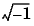MATLAB Function Referencecomplex

Construct complex data from real and imaginary components

Syntax

• ```c = complex(a,b)
c = complex(a)
```

Description

```c = complex(a,b) ``` creates a complex output, `c`, from the two real inputs.

• ```c = a + bi
```

The output is the same size as the inputs, which must be scalars or equally sized vectors, matrices, or multi-dimensional arrays.

 Note    If `b` is all zeros, `c` is complex and the value of all its imaginary components is `0`. In contrast, the result of the addition `a+0i` returns a strictly real result.

The following describes when `a` and `b` can have different data types, and the resulting data type of the output `c`:

• If either of `a` or `b` has type `single`, `c` has type `single`.
• If either of `a` or `b` has an integer data type, the other must have the same integer data type or type scalar `double`, and `c` has the same integer data type.

`c = complex(a)` for real `a` returns the complex result `c` with real part `a` and `0` as the value of all imaginary components. Even though the value of all imaginary components is `0`, `c` is complex and `isreal(c)` returns false.

The `complex` function provides a useful substitute for expressions such as

• ```a + i*b   or   a + j*b
```

in cases when the names "`i`" and "`j`" may be used for other variables (and do not equal), when `a` and `b` are not `single` or `double`, or when `b` is all zero.

Example

Create complex `uint8` vector from two real `uint8` vectors.

• ```a = uint8([1;2;3;4])
b = uint8([2;2;7;7])
c = complex(a,b)
c =
1.0000 + 2.0000i
2.0000 + 2.0000i
3.0000 + 7.0000i
4.0000 + 7.0000i
```

See Also

`abs`, `angle`, `conj`, `i`, `imag`, `isreal`, `j`, `real`

© 1994-2005 The MathWorks, Inc.